Chapter 11, Problem 98AE

Chapter
Section
Textbook Problem

# For each of the following solute-solvent combinations, state the sign and relative magnitudes for ∆H1, ∆H2, ∆H3, and ∆Hsoln, (as defined in Fig. 10-1 of the text). Explain your answers.a.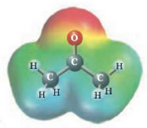andb.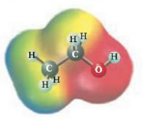and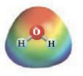c.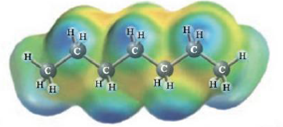and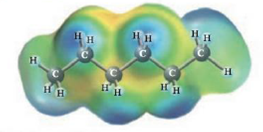d.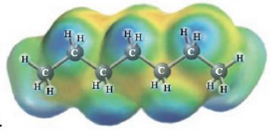and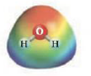a)

Interpretation Introduction

Interpretation: The dissolution of the given following solute and solvent has to be explained.

Concept Introduction: Concept introduction:

Raoult's law:

The mole fraction of a solute is related to the vapor pressure of the solution thus,

Psolution=P°solventXsolvent......(1)Pisvapor pressureof the solutionsolventpressureof the solventXsolvent mole fraction ofsolvent

Explanation

To find the Acetone and water polarity

CH3COCH3 and H2O

The electrostatic possible drawing illustrates that acetone ( CH3COCH3 ), like water, is a polar substance (each has a red end indicating the partial negative end of the dipole moment and a blue end indicating the partial positive end). For a polar solute in a polar solvent, ΔH1 and ΔH2 will be large and positive, whilst ΔH3 will be a large negative value

b)

Interpretation Introduction

Interpretation: The dissolution of the given following solute and solvent has to be explained.

Concept Introduction: Concept introduction:

Raoult's law:

The mole fraction of a solute is related to the vapor pressure of the solution thus,

Psolution=P°solventXsolvent......(1)Pisvapor pressureof the solutionsolventpressureof the solventXsolvent mole fraction ofsolvent

c)

Interpretation Introduction

Interpretation: The dissolution of the given following solute and solvent has to be explained.

Concept Introduction: Concept introduction:

Raoult's law:

The mole fraction of a solute is related to the vapor pressure of the solution thus,

Psolution=P°solventXsolvent......(1)Pisvapor pressureof the solutionsolventpressureof the solventXsolvent mole fraction ofsolvent

d)

Interpretation Introduction

Interpretation: The dissolution of the given following solute and solvent has to be explained.

Concept Introduction: Concept introduction:

Raoult's law:

The mole fraction of a solute is related to the vapor pressure of the solution thus,

Psolution=P°solventXsolvent......(1)Pisvapor pressureof the solutionsolventpressureof the solventXsolvent mole fraction ofsolvent

### Still sussing out bartleby?

Check out a sample textbook solution.

See a sample solution

#### The Solution to Your Study Problems

Bartleby provides explanations to thousands of textbook problems written by our experts, many with advanced degrees!

Get Started